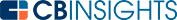# What is the Present Value Formula?The present value formula is a tool investors and business owners use to estimate if an investment made today for a given rate of return will be worth the money they put into it.

It helps you determine how much you can gain from an investment at a given rate of return after a specified time.

Before diving into the present value formula itself, it’s important to understand the theory behind it — the time value of money. It’s an economic theory that describes how money promised for the future is less valuable than money in hand right now.

It’s the theory behind interest payments, which make it worth your while to invest money in anticipation of future gains or, for that matter, why a bank charges you interest for lending you money. Simply put, you can’t spend money you don’t have, so if it’s going to sit around somewhere else, it better be worth it.

Here’s what the formula looks like:

Present value (PV) = C / (1 + r)^n

Where:

C = the future return or cash flow
r = the rate or return or the discount rate

n = is the number of periods

N.B. n is an exponent, meaning it tells you the number of times you’ll do this division. For example, to calculate the present value of \$1,000 you expect to receive in three years, assuming a discount rate of 5%, the formula would look like this:

Present Value = \$1,000 / 1.05 / 1.05 / 1.05

Which gives a present value of \$863.84.

The discount rate could also be an interest rate. Say you wanted to end up with \$1,000 after a three-year investment earning 5%. With this formula, you would know you’d need to invest about \$864 now.

More complicated versions of the formula allow you to figure out the present value of money when the expected cash flow varies from year to year.

## Understanding the discount rate

What is the discount rate and how is it determined? One discount rate you might hear about in the news is the discount rate charged by the Federal Reserve, when lending money to its member banks to meet cash reserve requirements. The Federal Reserve sets this rate with an eye on the overall US economy.

For example, according to TheBalance.com, it lowered its discount rate to 0.25% in March 2020 to make it cheaper for banks to stay liquid in the face of economic disruptions to come as Covid-19 became a global pandemic.

But this is not the discount rate investors look at. Investors are interested in things like cash flow and the cost of capital that a business or startup is paying for. A very simple example in corporate finance is that the discount rate is equal to the interest rate a company will pay to borrow the money to fund a new project.

But debt might not be the only source of capital available to a business; if it’s a public company with stocks paying out dividends, you also must factor in that obligation to shareholders.

One commonly used formula for determining the discount rate is the weighted average cost of capital, or WACC, with 8 different components such as the market value of the firm’s equity, the value of its debt, the cost of its debt, and even its tax rate.

But that’s not the only method. Some models use an investor’s required rate of return as the discount rate (i.e., how much of a gain they want to realize). Some factors an investor might consider are the volatility of the startup’s cash flow, the quality of its leadership, or the uniqueness of its product, among many others.

Financial services powerhouse Deloitte notes that:

“The discount rate … can be tricky to get right [and] estimated discount rates may vary between practitioners.”

The present value formula is a way to understand the required investment today to achieve a specific value or gain at a point in the future at a specific rate of return. In other words, it calculates how much you need to invest to have your desired sum in the future.

You may also like# www.cbinsights.com